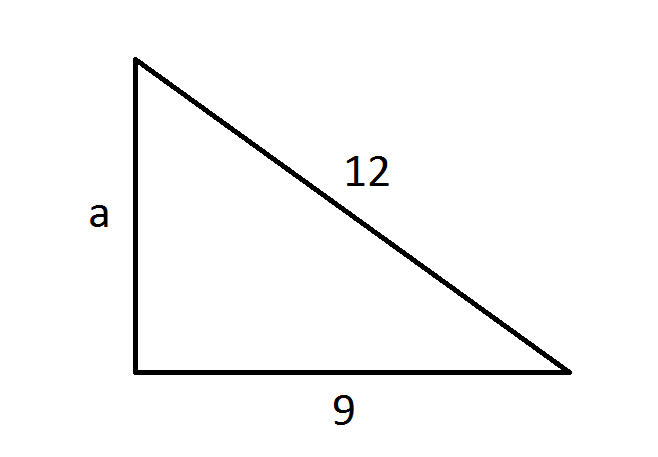HAMS Unit 2 Progression 1
starstarstarstarstarstarstarstarstarstar
by Geoffrey Kirnon
| 9 Questions
1
1
Looking at the right triangle below, what is the length of the missing side? Round to the nearest tenth.

3.7
10
100
1000002
1
Looking at the right triangle above, what is the length of the missing side? Round to the nearest tenth.
7.9
15
63
225
3
3
Which of the following is a right triangle?
leg 1 = 3, leg 2 = 4, hypotenus = 5
leg 1 = 12, leg 2 = 16, hypotenus = 20
leg 1 = 8 , leg 2 = 8, hypotenus = 20
leg 1 = 8, leg 2 = 15, hypotenus = 17
leg 1 = 4, leg 2 = 5, hypotenus = 10
4
1
The bottom of a ladder must be placed 9 feet from a wall. The ladder is 15 feet long. How far above the ground
does the ladder touch the wall? Round to the nearest whole number.
12 inches
12 feet
17 feet
17 inches
5
1
A triangle is an isosceles triangle with legs that measure 17 inches and a base of 30 inches. What is the height of triangle ABC?
8 inches
15 inches
24 inches
47 inches
6
1
On the coordinate plane, which point is the greatest distance from the origin?
A. (-7, -2)
B. (-7, 1)
C. ( 4, 6)
D. ( 6, 4)
7
1
On the coordinate plane, rectangle FGHI has the following vertices:

F ( -2, 4)
G (-2, -2)
H ( 6, 4)
I ( 6, -2)

What is the length of the diagonal of rectangle FHGI?
A. 7 units

B. 9 units
C. 10 units
D. 14 units
8
3
This Question has more than one correct answer. Select ALL of the answer choices that are correct. Lawrence constructed a right triangle using three drinking straws. Which of the straw length sets below could he use to form a right triangle?
A. 2 cm, 5 cm, 7 cm

B. 6 cm, 8 cm, 10 cm
C. 5 cm, 12 cm, 13 cm
D. 5 cm, 12 cm, 15 cm
E. 10 cm, 10 cm, 20 cm
9
1
Constructed Response Item
5. Romeo needs to see the love of his life, Juliet. The only open window is on the second floor, 14 feet above the ground. Romeo will need to borrow a ladder from one of Juliet’s neighbors. There is a bush along the edge of her house, so Romeo will have to place the ladder 10 feet from the house. If ladders are available in maximum lengths of 17 feet or 19 feet which ladder should Romeo purchase in order to reach the window?